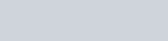ChemTalk

# Carnot Cycle

## Core Concepts

This article will cover what the Carnot cycle is, the states and thermodynamic processes it comprises, the efficiency of the Carnot cycle, refrigeration, and the diagrams that are used to represent it. After reading this article, you will be able to understand and describe the uses and applications of the Carnot cycle, in addition to what is shown in relevant diagrams.

## The Carnot Cycle

The Carnot Cycle is a theoretical thermodynamic cycle that involves a reversible changes to an ideal gas. Furthermore, it is the ideal model of a thermodynamic system for a heat engine. The cycle comprises four states and we define the transitions between these states as processes labeled I, II, III, and IV. Additionally, there are two binding isotherms, one at high temperature, Th, and one at low temperature, Tc. These isotherms go from state to state in which a full cycle is completed.

#### Process I: Isothermal Expansion

Process I is an isothermal gas expansion which means that temperature remains constant as the volume of the gas increases. The ideal gas of the system absorbs some amount of heat, qin, at a high temperature, Th, and expands. In this process, the gas does work on the surroundings as it expands. Due to how temperature remains constant as the gas expands, no change in internal energy occurs during this process.Process I of the Carnot cycle, shown by an ideal gas-pistol model.

The second step is an adiabatic gas expansion which means that no heat transfer occurs. Here, the system is thermally insulated as gas continues to expand and do work on the surroundings. As a result, the system cools to a lower temperature, Tc.Process II of the Carnot cycle, shown by an ideal gas-piston model.

#### Process III: Isothermal Compression

Following the adiabatic expansion is an isothermal gas compression. In this process, the surroundings now instead do work on the gas at Tc. This results in a loss of heat, qout.Process III of the Carnot cycle, shown by an ideal gas-piston model.

The final step is an adiabatic gas compression. In this case, the system is once again thermally insulated and the surroundings continue to do work on the gas. This results in the temperature rising back to Th.Process IV of the Carnot cycle, shown by an ideal gas-piston model.

## Efficiency of the Carnot Cycle

The Carnot cycle has the greatest possible efficiency for an engine. The ratio of energy output to energy input defines Carnot engine efficiency. Therefore, the efficiency of the Carnot engine would be equivalent to the net work done divided by the heat absorbed by the system. This is true for all reversible engines. However, modelling a thermodynamic cycle as a Carnot cycle assumes that processes such as friction are not present and that there is no conduction of heat between different parts of an engine at different temperatures.

Below are relevant formulas for efficiency:## Carnot Cycle Refrigeration

As a reversible cycle, all four processes of the Carnot cycle can be reversed which reverses the direction of heat and work. The result is what is known as a refrigeration cycle. The primary difference between a refrigeration cycle and the Carnot cycle is their objectives. A refrigeration cycle begins with an isothermal heat transfer from a cold medium to a refrigerant. Next is isentropic (reversible adiabatic) compression followed by isothermal heat rejection. Finally, isentropic expansion occurs to complete the cycle.

## P-V Diagram

Below is a P-V, or pressure-volume, diagram of the Carnot cycle. This diagram plots the change in pressure with respect to volume for each of the four processes. Additionally, it shows that there is no net change in the state of the system with the completion of the cycle in which it returns to starting pressure and volume. Also worth noting is that the area bounded by the complete cycle path represents the total work done during a cycle.

## T-S Diagram

Below is a T-S diagram, or temperature-entropy diagram, of the Carnot cycle. This diagram plots the change in temperature with respect to entropy for each of the four processes, allowing for the visualization of changes in temperature and entropy during a complete cycle. The plot appears as a rectangle, the area of which represents total heat transfer. Also worth noting is that the T-S diagram shows that processes I and III are isothermal as there is no change in temperature. Furthermore, there is no change in entropy for processes II and IV which means that there is no heat transfer, showing that they are adiabatic.

## Practice Problems

1. A Carnot engine is operated at 60% efficiency and it exhausts heat into a heat sink at 298 K. In order to increase the efficiency of the engine to 80%, what temperature would the heat reservoir have to be raised to?
2. A Carnot engine absorbed 1.5 kJ of heat at 300 K and exhausted 500 J of heat at the end of the cycle. What is the final temperature?
3. An indoor heater that operates on the Carnot cycle heats up a building at a rate of 60 kJ to maintain an indoor temperature of 25 degrees Celsius. How much energy is used to operate the heater if the temperature outdoors is 0 degrees Celsius?

1. 1490 K
2. 100 K
3. 715.2 kJ